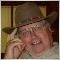# How to calculate free margin left before opening an order ?[Deleted]

Hello,

I would like to know the expression to calculate :

In my EA I would make something like this :

- I try 1 lot

- If the free margin is not ok, I try 0.9 lots

- If the free margin is not ok, I try 0.8 lots

- and continue until have the minimum lots to open an order

Have you got an expression to make this ?

Thank you...591

nazoreen:

Hello,

I would like to know the expression to calculate :

In my EA I would make something like this :

- I try 1 lot

- If the free margin is not ok, I try 0.9 lots

- If the free margin is not ok, I try 0.8 lots

- and continue until have the minimum lots to open an order

Have you got an expression to make this ?

Thank you...

https://docs.mql4.com/account/AccountFreeMargin

```double Risk=3;  // percent of free margin to calculate lot size.

double Lots=NormalizeDouble(AccountFreeMargin()*Risk/100/1000.0,1);   // Standard lot size```[Deleted]

Hello and thank you...

Could you explain numbers "100" and "1000" ?!?

Thanks a lot ![Deleted]

Hello,